# Texas Go Math Grade 4 Lesson 17.2 Answer Key Use Frequency Tables

Refer to our Texas Go Math Grade 4 Answer Key Pdf to score good marks in the exams. Test yourself by practicing the problems from Texas Go Math Grade 4 Lesson 17.2 Answer Key Use Frequency Tables.

## Texas Go Math Grade 4 Lesson 17.2 Answer Key Use Frequency Tables

Essential Question

How do you solve problems using a frequency table?
Answer: A frequency table problem is one where the number of times an observation occurs. It is recorded in an experiment or study is known. You could use a frequency table to find out the most common numbers in a set.
Example:
Using the key: H = Hockey, B = Badminton, F = Football, each letter below indicates a student joining the sports club.
H H B F B H F F H B
B B H F H H F B H B
a) Construct a frequency table for the data.
b) Determine which club has the highest frequency and which club has the lowest frequency.
c) Calculate the percentage of students who joined the Hockey club.
Solution:
a) First, we tally the student count by the clubs they belong to. The total number of counts each club receives is the frequency of the number of students joining that club. We organize the data in the frequency table.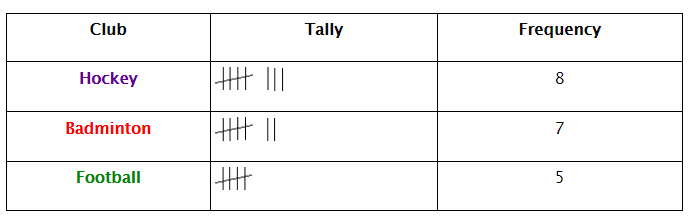b) From the frequency table, we can determine which club has the highest frequency and which club has the lowest frequency. Hockey is the club with the highest frequency and football is the club with the lowest frequency.
c) Percentage of students who joined the Hockey club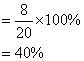Unlock the Problem

Example 1

Michael asked his friends the weight of their small dogs. He recorded the frequency of each weight in a frequency table. How many of Michael’s friends have dogs that weigh 9 pounds?Think: The number in the frequency column is the number of friends who said they have4dogs that weigh the weight listed in the Weight column.

Michael has ___________ friends who have dogs that weigh 9 pounds.
Michael has 3 friends who have dogs that weigh 9 pounds.

How many more of Michael’s friends have dogs that weigh 12 and 13 pounds than friends with dogs that weigh 10 and 11 pounds? ____________
Michael’s friends have dogs that weigh 12 and 13 pounds are 4, 2=4+2=6.
Friends with dogs that weigh 10 and 11 pounds=0,1=0+1=1.
5 more dogs are there that weigh 12 and 13. (6-1=5).

Example 2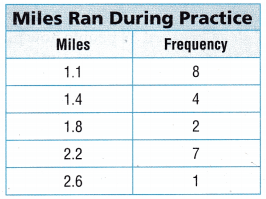To train for a cross country track meet, Dylan and his teammates practised running through the woods. Dylan’s coach recorded the distances that they ran in a frequency table. How many of Dylan’s teammates ran more than 2 miles?

Think: Add the number in the frequency column for the students who ran more than 2 miles.

_________________ of Dylan’s teammates ran more than 2 miles during practice.8 of Dylan’s teammates ran more than 2 miles during practice.

• How many of Dylan’s teammates ran during practice? _________________
Add all the frequencies of the frequency column then we will get the number of teammates.
The number of teammates ran during practice=8+4+2+7+1+=22.
There are 22 teammates who ran during practice.

• how many more teammates ran 1.1 miles and 2.2 miles than ran 1.4, 1.8, or 2.6 miles combined? _________________
The number of teammates ran during the practice of mile 1.1 is 8
The number of teammates ran during the practice of mile 2.2 is 7
The number of teammates ran during the practice of mile 1.4 is 4
The number of teammates ran during the practice of mile 1.8 is 2
The number of teammates ran during the practice of mile 2.6 is 1
The teammates ran during the practice of miles 1.4, 1.8, 2.6 combines=4+2+1=7.
The teammates ran during the practice of miles 1.1, 2.2 combines 8+7=15
The difference is 15-7=8
8 more teammates ran 1.1 and 2.2 miles than the other 1.4, 1.8, 2.6

Example 3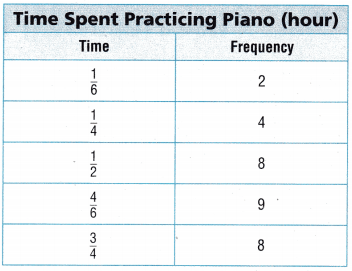Brendan’s mom recorded the amount of time Brendan spent practicing the piano every day for 1 month. Which amount of time did Brendan practice the most?

Think: Look for the greatest number in the frequency column to determine which amount of time he spent practicing the most.

Brendan practiced the piano most often for ___________ of an hour.
Brendan practised the piano most often for 1/6 of an hour. Because it is having the least frequency of 2.
• Did Brendan spend more days practising the piano more than $$\frac{1}{2}$$ hour or less than $$\frac{1}{2}$$ hour?
Yes, he spends more time practising the piano. He spends more than 1/2 an hour. The time he spend was 4/6 of an hour and it is having the highest frequency of 9. Sometimes he spends very often less than 1/2 an hour and the timings were 1/6, 1/4 which are having frequencies of 2 and 4.

• Which two times did Brendan practice the least amount of times?
1/6, 1/4 are the two times Brendan practice the least amount of times. Because their frequencies are low 2 and 4. By checking the frequencies we can determine according to the question.

Share and Show

Use the frequency table for 1-3.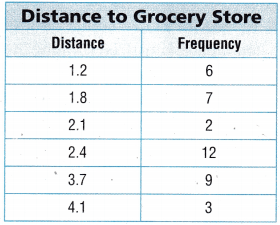Question 1.
Reagan asked 39 people how many miles they lived from the closest grocery store. She recorded their answers in a frequency table. How many people live 1.8 miles or 3.7 or more miles from the grocery store?
There are three people who live more than 3.7 miles. And the highest mile was 4.1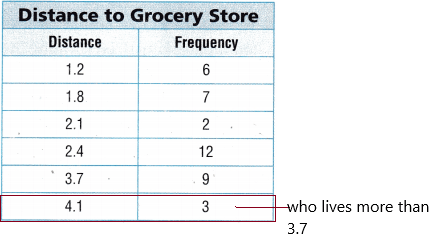The number of people that live 1.8 miles from the store is ______________ .
Answer: There are 7 people that live 1.8 miles from the store.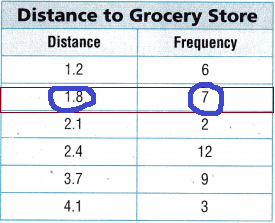The number of people that live 3.7 or more miles from the store is ______________ .
There are 9 people who live 3.7 miles from the store.
There are 3 people who live 4.1 miles from the store.

______________ + ______________ = ______________; ______________ people live 1.8 miles or 3.7 or more miles from the store.
7+12=19.   (3.7 or more miles:9+3=12).
19 people live 1.8 miles or 3.7 miles or more miles from the store.

How many people live less than 2.4 miles from the grocery store?Add all the frequencies present in the frequency column which are below 2.4 miles that are 1.2, 1.8, 2.1 and their frequencies are 6, 7, 2.
15 people living less than 2.4 miles from the grocery store.

Question 3.
How many people drive more than 8 miles to the grocery store and back?
3 people drive more than 8 miles to the grocery store and back.In the question asked driving to the store and back, both actions are required here. Forgoing to the store is 4.1 miles and from coming back to the store is also the same miles that are 4.1.
4.1+4.1=8.2 miles. That’s why the frequency is 3.

Problem Solving

Use the frequency table for 4-6.Question 4.
Jake asked a group of friends he hikes with how many miles they hiked during their vacation How many of Jake’s friends hiked at least 2$$\frac{1}{4}$$ miles during their vacation?Explanation:
Jake’s friends hiked at least 2$$\frac{1}{4}$$ miles during their vacation is 15 members.

Question 5.H.O.T. Multi-Step How many more friends hiked 3 miles or less than friends that hiked 3$$\frac{1}{2}$$ miles or more? Explain your answer
The number of friends hiked 3 miles are 7
The number of friends hiked 3$$\frac{1}{2}$$ miles are 11.
Now the given question is to find out the number of more friends hiked 3 miles or less than 3$$\frac{1}{2}$$ miles.
Subtract the frequencies 11 and 7 then we get to know the answer.
11-7=4.
There are 4 more friends who hiked 3 miles and less than 3$$\frac{1}{2}$$ miles.

Use Math Language Explain how you would find the number of Jake’s friends that hiked between 2$$\frac{1}{4}$$ and 4$$\frac{3}{4}$$ miles.
The number of Jake’s friends that hiked 2$$\frac{1}{4}$$ miles=15
The number of Jake’s friends that hiked 4$$\frac{3}{4}$$ miles=3
The given question is to find out the number of Jake’s friends that hiked between 2$$\frac{1}{4}$$ and 4$$\frac{3}{4}$$ miles.
If we add both frequencies then we get to know the number of friends that hiked between 2 1/4 and 4 3/4=15+3=18.
18 people who hiked between 2$$\frac{1}{4}$$ and 4$$\frac{3}{4}$$ miles.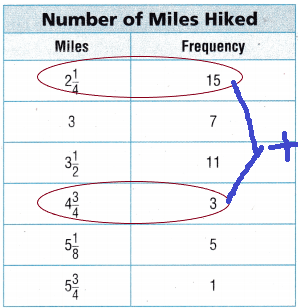Use the frequency table for 7-8.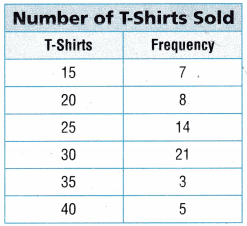Question 7.
Multi-Step During a fund-raiser, students were asked to sell t-shirts. The school recorded the number of students who sold t-shirts in a frequency table. How many students sold more than 20 t-shirts?
The students who sold more than 20 t-shirts=’X’.
Add all the frequencies of t-shirts who are more than 20 t-shirts:
14+21+3+5=43.
There are 43 students who sold more than 20 t-shirts.
X=43.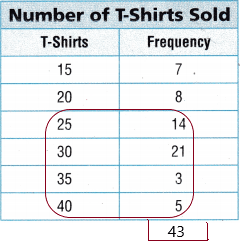Question 8.
H.O.T. Apply How many t-shirts did the most number of students sell? How many total t-shirts were sold by those students? Explain.
The t-shirts most students sell means we need to check the frequencies first. The greatest frequencies are 14 and 21.
The 14 students sold 25 t-shirts.
The 21 students sold 30 t-shirts.
add the t-shirts column which is having with high frequencies that are 25+30=55.
There are 55 t-shirts sold by those students.Multi-Step Monica recorded the frequency of the number of students and the length of time it took them to play a math game. How many more students took $$\frac{1}{2}$$ hour to play the game than the students that took $$\frac{3}{4}$$ hour and 1 hour combined?(A) 17
(B) 6
(C) 20
(D) 1Explanation:
The students took $$\frac{1}{2}$$ hour to play the game=18
The students that took $$\frac{3}{4}$$ hour and 1 hour combined=8+9=17.
We need to find out more students took $$\frac{1}{2}$$ hour to play the game than the students that took $$\frac{3}{4}$$ hour and 1 hour combined. If we subtract then we get the answer.
18-17=1.
1 student more took 1/2 an hour to play a game.

Use the Age of Chorus Members frequency table for 10-11.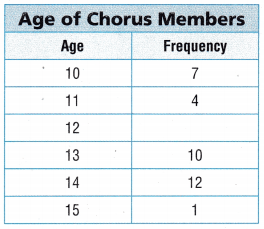Question 10.
There are half as many 12 year old chorus members as there are 14 year olds. How many 12 year old chorus members are there?
(A) 2
(B) 10
(C) 6
(D) 8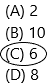Explanation:
The 14-year-old chorus members are 12.
The 12-year-old chorus members are 6 because there is half of the 14-year-old means half of 12. This can be written as:
12/2=6.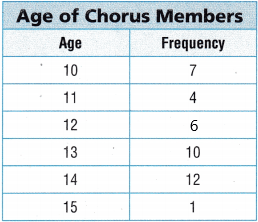Question 11.
Multi-Step How many fewer 13 year olds are there in chorus than 10 year olds and 14 year olds combined?
(A) 19
(B) 9
(C) 15
(D) 16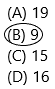Explanation:
The number of 13-year-old chorus members=10
The number of 10-year-old and 14-year-old chorus members combined=12+7=19.
Compared to 10 and 14-year-old chorus members how many members are low in 13-year-old chorus members? If we subtract then we get the answer.
19-10=9.

TEXAS Test Prep

Dafnie and her friends like to ride bikes. How many of Dafnie’s friends rode their bikes either less than 1.1 miles or more than 2.0 miles?(A) 23
(B) 24
(C) 11
(D) 12Explanation: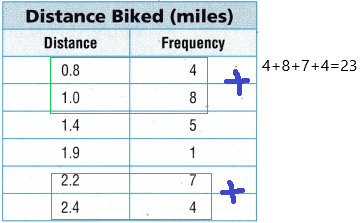add all frequencies less than 1.1 distance and more than 2.0 miles then we get the answer:4+8+7+4=23.

### Texas Go Math Grade 4 Lesson 17.2 Homework and Practice Answer Key

Use the frequency table for 1-4.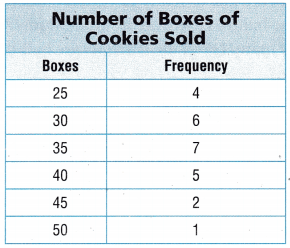Question 1.
Kayla is in charge of cookie sales for her scout troop. How many members sold more than 40 boxes of cookies?
Add all the frequencies which are having more than 40 cookies:2+1 =3.
3 members sold more than 40 boxes of cookies. This will be represented in the below diagram:Go Math Lesson 17.2 4th Grade Frequency Table Problems Question 2.
How many members sold fewer than 40 boxes of cookies?
17 members sold less than 40 boxes of cookies. This will be represented in the below diagram: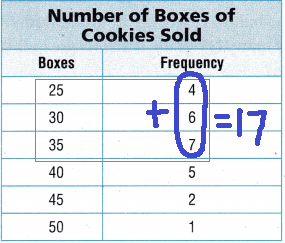Question 3.
How many boxes of cookies did the most in number of members sell?
50 boxes of cookies are sold by 1 member.Question 4.
How many members are represented Kayla’s frequency table?
add all the frequencies in the frequency column to get the number of members:4+6+7+5+2+1=25.
25 members are represented Kayla’s frequency table.

Problem Solving

Use the frequency table for 5-7.Question 5.
The table shows the number of absences in the fourth grade during the school year. How many students were absent either 1 or 2 times during the school year?
25 students are absent 1or 2 times during the school year.
add the frequencies of 1 and 2=10+15=25.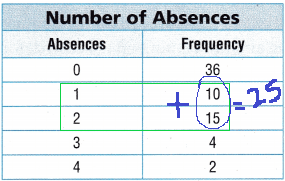How many students were absent more than 2 times during the school year?
6 students are absent more than 2 times.
add all the frequencies of 3 and 4: 4+2=6Question 7.
Multi-Step How many more students were absent 2 days or less than students who were absent 3 days or more? Explain your answer.
9 more students were absent.
The students were absent 2 days=15
The students were absent 3 or more days=4+2=6
If we subtract then we get answer:15-6=9.

Lesson Check

Use the Grade of Marching Band Members frequency table for 8-9.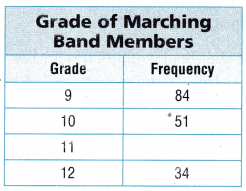Question 8.
There are half as many 11th grade students in the band as there are 9th grade students. How many 11th grade students are in the band?
(A) 42
(B) 84
(C) 51
(D) 17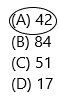Explanation:
The frequency of 9th-grade students are 84
The half of 11th-grade students in the band as there are 9th grade students=84/2=42.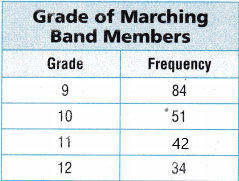Question 9.
How many more 9th grade students are in the band than 10th gade students?
(A) 50
(B) 9
(C) 33
(D) 51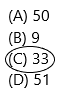Explanation:
The number of 9th grades students=84
The number of 10th grade students=51.
Subtract these two frequencies to know the more 9th grade students are in the band than 10th grade students=84-51=33

Use the April Rainfall frequency table for 10 – 11.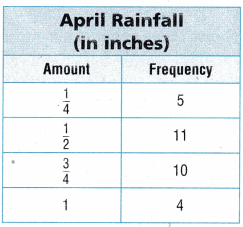Multi-Step Olivia recorded the frequency of the amount of rain that fell each day in April. How many more days did it rain less than an inch than it rained 1 inch?
(A) 4 days
(B) 10 days
(C) 26 days
(D) 22 days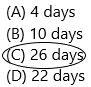Explanation:
The question asked was more days did it rain less than an inch than it rained 1 inch. So add all the frequencies of less than an inch.
5+11+10=26 days.

Question 11.
Multi-Step How many fewer days did it rain $$\frac{1}{4}$$ inch than $$\frac{1}{2}$$ inch and $$\frac{3}{4}$$ inch combined?
(A) 6 days
(B) 16 days
(C) 26 days
(D) 4 daysThe number of days did it rain $$\frac{1}{4}$$ inch=5
The number of days did it rain $$\frac{1}{2}$$ inch and $$\frac{3}{4}$$ inch combined:11+10=21
The number of fewer days did it rain $$\frac{1}{4}$$ inch than $$\frac{1}{2}$$ inch and $$\frac{3}{4}$$ inch combined: